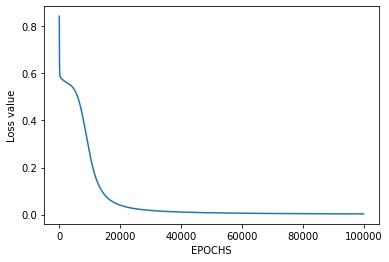# Implementation of Artificial Neural Network for NOR Logic Gate with 2-bit Binary Input

• Last Updated : 28 Jul, 2021

Artificial Neural Network (ANN) is a computational model based on the biological neural networks of animal brains. ANN is modeled with three types of layers: an input layer, hidden layers (one or more), and an output layer. Each layer comprises nodes (like biological neurons) are called Artificial Neurons. All nodes are connected with weighted edges (like synapses in biological brains) between two layers. Initially, with the forward propagation function, the output is predicted. Then through backpropagation, the weight and bias to the nodes are updated to minimizing the error in prediction to attain the convergence of cost function in determining the final output.

NOR logical function truth table for 2-bit binary variables, i.e, the input vectorand the corresponding output001
010
100
110

Approach:
Step1: Import the required Python libraries
Step2: Define Activation Function : Sigmoid Function
Step3: Initialize neural network parameters (weights, bias)
and define model hyperparameters (number of iterations, learning rate)
Step4: Forward Propagation
Step5: Backward Propagation
Step6: Update weight and bias parameters
Step7: Train the learning model
Step8: Plot Loss value vs Epoch
Step9: Test the model performance

Python Implementation:

 `# import Python Libraries``import` `numpy as np``from` `matplotlib ``import` `pyplot as plt`` ` `# Sigmoid Function``def` `sigmoid(z):``    ``return` `1` `/` `(``1` `+` `np.exp(``-``z))`` ` `# Initialization of the neural network parameters``# Initialized all the weights in the range of between 0 and 1``# Bias values are initialized to 0``def` `initializeParameters(inputFeatures, neuronsInHiddenLayers, outputFeatures):``    ``W1 ``=` `np.random.randn(neuronsInHiddenLayers, inputFeatures)``    ``W2 ``=` `np.random.randn(outputFeatures, neuronsInHiddenLayers)``    ``b1 ``=` `np.zeros((neuronsInHiddenLayers, ``1``))``    ``b2 ``=` `np.zeros((outputFeatures, ``1``))``     ` `    ``parameters ``=` `{``"W1"` `: W1, ``"b1"``: b1,``                  ``"W2"` `: W2, ``"b2"``: b2}``    ``return` `parameters`` ` `# Forward Propagation``def` `forwardPropagation(X, Y, parameters):``    ``m ``=` `X.shape[``1``]``    ``W1 ``=` `parameters[``"W1"``]``    ``W2 ``=` `parameters[``"W2"``]``    ``b1 ``=` `parameters[``"b1"``]``    ``b2 ``=` `parameters[``"b2"``]`` ` `    ``Z1 ``=` `np.dot(W1, X) ``+` `b1``    ``A1 ``=` `sigmoid(Z1)``    ``Z2 ``=` `np.dot(W2, A1) ``+` `b2``    ``A2 ``=` `sigmoid(Z2)`` ` `    ``cache ``=` `(Z1, A1, W1, b1, Z2, A2, W2, b2)``    ``logprobs ``=` `np.multiply(np.log(A2), Y) ``+` `np.multiply(np.log(``1` `-` `A2), (``1` `-` `Y))``    ``cost ``=` `-``np.``sum``(logprobs) ``/` `m``    ``return` `cost, cache, A2`` ` `# Backward Propagation``def` `backwardPropagation(X, Y, cache):``    ``m ``=` `X.shape[``1``]``    ``(Z1, A1, W1, b1, Z2, A2, W2, b2) ``=` `cache``     ` `    ``dZ2 ``=` `A2 ``-` `Y``    ``dW2 ``=` `np.dot(dZ2, A1.T) ``/` `m``    ``db2 ``=` `np.``sum``(dZ2, axis ``=` `1``, keepdims ``=` `True``)``     ` `    ``dA1 ``=` `np.dot(W2.T, dZ2)``    ``dZ1 ``=` `np.multiply(dA1, A1 ``*` `(``1``-` `A1))``    ``dW1 ``=` `np.dot(dZ1, X.T) ``/` `m``    ``db1 ``=` `np.``sum``(dZ1, axis ``=` `1``, keepdims ``=` `True``) ``/` `m``     ` `    ``gradients ``=` `{``"dZ2"``: dZ2, ``"dW2"``: dW2, ``"db2"``: db2,``                 ``"dZ1"``: dZ1, ``"dW1"``: dW1, ``"db1"``: db1}``    ``return` `gradients`` ` `# Updating the weights based on the negative gradients``def` `updateParameters(parameters, gradients, learningRate):``    ``parameters[``"W1"``] ``=` `parameters[``"W1"``] ``-` `learningRate ``*` `gradients[``"dW1"``]``    ``parameters[``"W2"``] ``=` `parameters[``"W2"``] ``-` `learningRate ``*` `gradients[``"dW2"``]``    ``parameters[``"b1"``] ``=` `parameters[``"b1"``] ``-` `learningRate ``*` `gradients[``"db1"``]``    ``parameters[``"b2"``] ``=` `parameters[``"b2"``] ``-` `learningRate ``*` `gradients[``"db2"``]``    ``return` `parameters`` ` `# Model to learn the NOR truth table ``X ``=` `np.array([[``0``, ``0``, ``1``, ``1``], [``0``, ``1``, ``0``, ``1``]]) ``# NOR input``Y ``=` `np.array([[``1``, ``0``, ``0``, ``0``]]) ``# NOR output`` ` `# Define model parameters``neuronsInHiddenLayers ``=` `2` `# number of hidden layer neurons (2)``inputFeatures ``=` `X.shape[``0``] ``# number of input features (2)``outputFeatures ``=` `Y.shape[``0``] ``# number of output features (1)``parameters ``=` `initializeParameters(inputFeatures, neuronsInHiddenLayers, outputFeatures)``epoch ``=` `100000``learningRate ``=` `0.01``losses ``=` `np.zeros((epoch, ``1``))`` ` `for` `i ``in` `range``(epoch):``    ``losses[i, ``0``], cache, A2 ``=` `forwardPropagation(X, Y, parameters)``    ``gradients ``=` `backwardPropagation(X, Y, cache)``    ``parameters ``=` `updateParameters(parameters, gradients, learningRate)`` ` `# Evaluating the performance``plt.figure()``plt.plot(losses)``plt.xlabel(``"EPOCHS"``)``plt.ylabel(``"Loss value"``)``plt.show()`` ` `# Testing``X ``=` `np.array([[``1``, ``1``, ``0``, ``0``], [``0``, ``1``, ``0``, ``1``]]) ``# NOR input``cost, _, A2 ``=` `forwardPropagation(X, Y, parameters)``prediction ``=` `(A2 > ``0.5``) ``*` `1.0``# print(A2)``print``(prediction)`

Output:

``````
```[[ 0.  0.  1.  0.]]
```

Here, the model predicted output for each of the test inputs are exactly matched with the NOR logic gate conventional output () according to the truth table and the cost function is also continuously converging.
Hence, it signifies that the Artificial Neural Network for the NOR logic gate is correctly implemented.

My Personal Notes arrow_drop_up